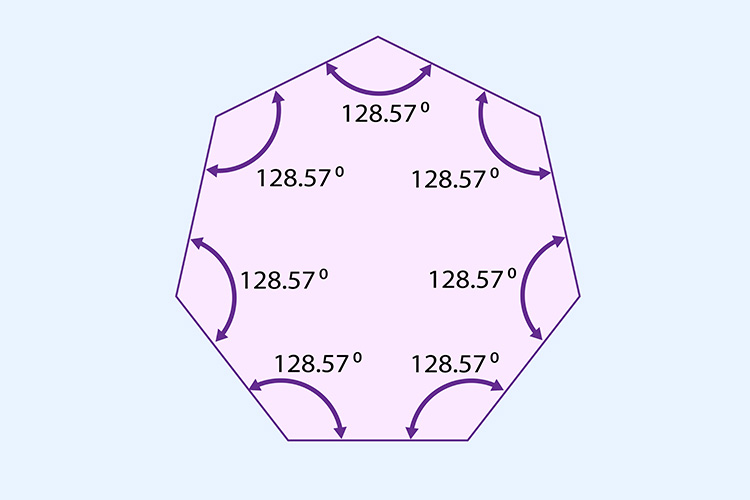# The total interior angles of a heptagon = 900°

Each time we add a side (triangle to square, square to pentagon, pentagon to hexagon) we add another 180°.

## Triangle to heptagon

 Triangle Square Pentagon Hexagon Heptagon 180° + 180° + 180° + 180° + 180° = 900°

The total internal angles of a heptagon = 900°Now divide the total internal angle by the number of corners.

i.e. divide 900° by 7.

900^circ/7=128.57^circ

 1 2 3 4 5 6 7 128.57° + 128.57° + 128.57° + 128.57° + 128.57° + 128.57° + 128.57° = 900°